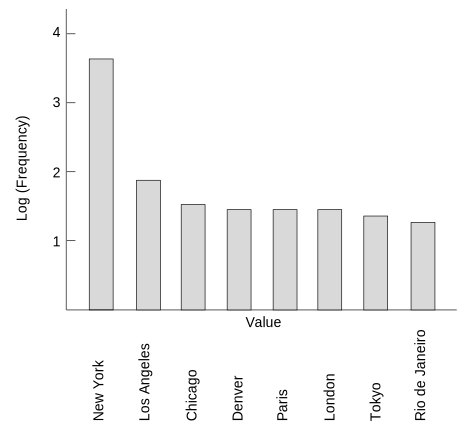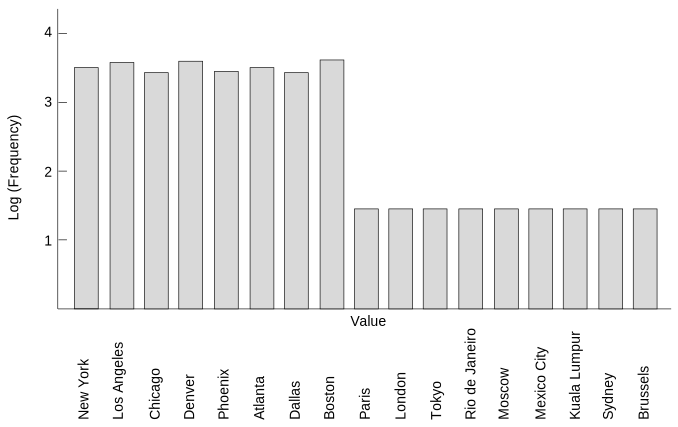16.10 - Maximum and Typical Column Value Frequencies - Teradata Database

Product
Release Number
16.10
Release Date
June 2017
Content Type
User Guide
Publication ID
B035-1094-161K
Language
English (United States)

This topic compares the concepts of maximum value and typical value and indicates methods for estimating the typical value for a column.

Variables are often distributed in such a way that they have no “typical” value, in which case the average, which represents the maximum likelihood value, is the only reasonable choice.

Each of these case studies examines different demographics for the same column. In each case, the data examined is for a City column.

Case Study 1

The following table indicates the number of distinct occurrences of City column values.

City Value Frequency of Value Log Frequency of Value
New York 4,000 3.602
Los Angeles 80 1.903
Chicago 35 1.544
Denver 30 1.477
Paris 30 1.477
London 30 1.477
Tokyo 25 1.398
Rio de Janeiro 20 1.301

The following histogram graphs the logarithm of the number of rows as a function of row values:The maximum value for this set is 4,000, but what is the typical value?

It is easy to see by visual inspection of the table above that the typical value for a variable is about 30. Note that the average value for this variable is 531.25, which is by no means typical of the values for the variable.

Maximum Value Typical Value
4,000 30

Case Study 2

The following table indicates the number of distinct occurrences of city column values.

City Value Frequency of Value Log Frequency of Value
New York 4,000 3.602
Los Angeles 4,000 3.602
Chicago 4,000 3.602
Denver 4,000 3.602
Paris 30 1.477
London 30 1.477
Tokyo 30 1.477
Rio de Janeiro 30 1.477

The following histogram graphs the logarithm of the number of rows as a function of row values:The maximum value for this set is 4,000, but what is the typical value?

It is impossible to determine a typical value for the scenario provided by this case history. When you encounter a situation like this, the optimum solution is to use the worst case as your typical value. In this case, that value is 4,000. Note that the average value for this variable is 2,015, which is not only not a typical value for the distribution of the variable, it is never a value for the variable in this case.

Maximum Value Typical Value
4,000 4,000

Case Study 3

The following table indicates the number of distinct occurrences of City column values.

City Value Frequency of Value Log Frequency of Value
New York 4,000 3.602
Los Angeles 4,100 3.613
Chicago 3,800 3.580
Denver 4,200 3.623
Phoenix 3,900 3.591
Atlanta 4,000 3.602
Dallas 3,800 3.580
Boston 4,150 3.618
Paris 30 1.477
London 30 1.477
Tokyo 30 1.477
Rio de Janeiro 30 1.477
Moscow 30 1.477
Mexico City 30 1.477
Kuala Lumpur 30 1.477
Sydney 30 1.477
Brussels 30 1.477

The following histogram graphs the logarithm of the number of rows as a function of row values:The maximum value for this set is 4,200, but what is the typical value?

It is impossible to determine an accurate “typical” value for the scenario provided by this case history. Like the scenario presented by Case Study 2, the distribution of values has two peaks at widely diverse points in the distribution. Unlike Case Study 2, the value set clustered around the value 4,000 is not constant.

When you encounter a situation like this, the optimum solution is to use a value around which the largest values cluster as your typical value. In this case, that value is 4,000.

Maximum Value Typical Value
4,200 4,000